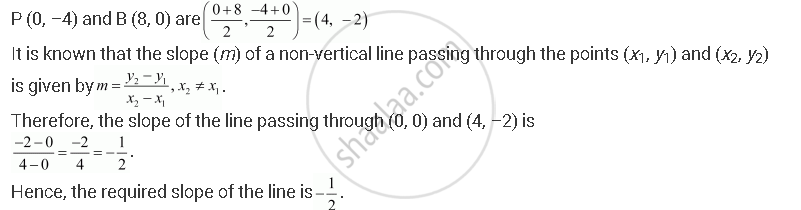CBSE (Arts) Class 11CBSE
Share

# Find the Slope of a Line, Which Passes Through the Origin, and the Mid-point of the Line Segment Joining the Points P (0, –4) and B (8, 0). - CBSE (Arts) Class 11 - Mathematics

#### Question

Find the slope of a line, which passes through the origin, and the mid-point of the line segment joining the points P (0, –4) and B (8, 0).

#### Solution

The coordinates of the mid-point of the line segment joining the pointsIs there an error in this question or solution?

#### Video TutorialsVIEW ALL 

Solution Find the Slope of a Line, Which Passes Through the Origin, and the Mid-point of the Line Segment Joining the Points P (0, –4) and B (8, 0). Concept: Slope of a Line.
S